# ExcelHow

This post will guide you how to sort IP address in your worksheet. How do I sort IP address with an excel formula. How to sort IP address with Text To Columns feature in excel.

## Sort IP Address with Formula

Assuming that you have an IP list of data in the range of cells B1:B5, and you want to sort those IP list from low to high, how to achieve the result with excel formula. You need to create a formula based on the Text Function, the LEFT function, the FIND function and the MID function, the RIGHT function. Just like this:

```=TEXT(LEFT(B1,FIND(".",B1,1)-1),"000") & "." & TEXT(MID(B1,FIND(
".",B1,1)+1,FIND(".",B1,FIND(".",B1,1)+1)-FIND(".",B1,1)-1),"000")
& "." & TEXT(MID(B1,FIND(".",B1,FIND(".",B1,1)+1)+1,FIND(".",B1,
FIND(".",B1,FIND(".",B1,1)+1)+1)-FIND(".",B1,FIND(".",B1,1)+1)-1),
"000") & "." & TEXT(RIGHT(B1,LEN(B1)-FIND(".",B1,FIND(".",B1,FIND(
".",B1,1)+1)+1)),"000")```

You will see this formula is quite long, but it is a single one formula and let’s see the detailed steps:

#1 type this formula into the formula box of Cell C1, then press Enter key in your keyboard, and drag the AutoFill Handler over other cells to apply this formula to sort other IP Address.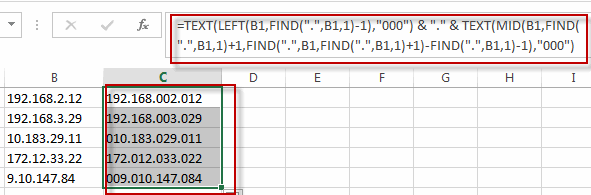#2 keep the newly IP Address selected, and go to DATA tab, click Sort A to Z under Sort&Filter group.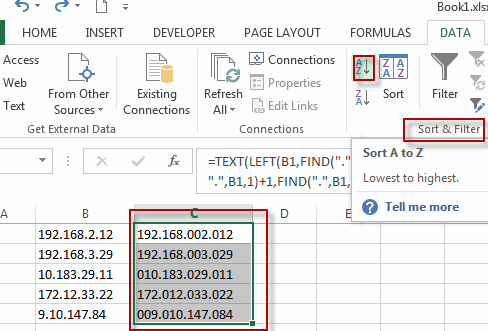#3 select Expand the selection radio button, and then click Sort button.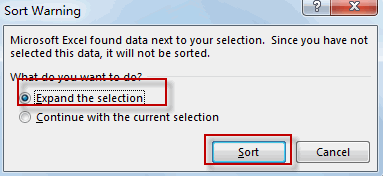#4 you will see the IP addresses have been sorted.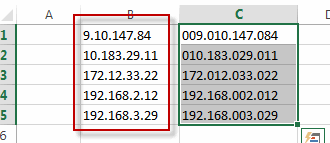## Sort IP Address with Text to Columns

You can also use the Text to Columns feature to sort IP address in excel, You can use this feature to divide the IP Addresses into separate columns and putting each octet in its own column. Before doing those actions, you need to make sure that there are three blank columns next to the right of your IP address. So just do the following steps:

#1 Select the cells that contain IP addresses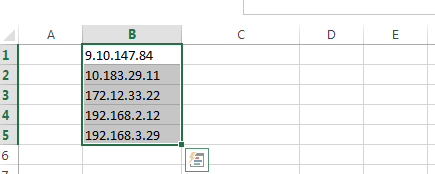#2 go to DATA tab, click Text to Columns command under Data Tools group. The Convert Text to Columns Wizard dialog will appear.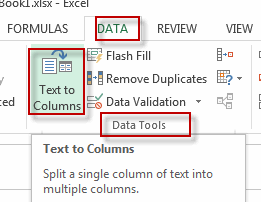#3 Checked Delimited option, and click Next button.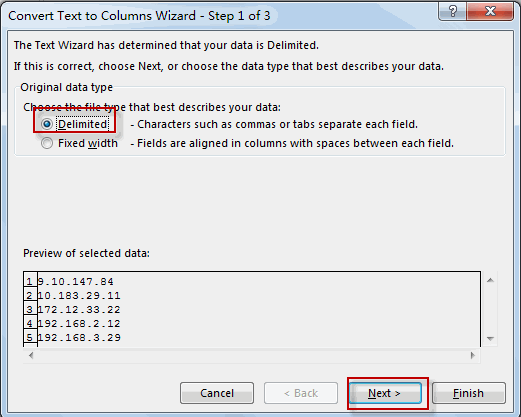#4 select the checkbox next to Other: box and then type . into the text box, and click Next button.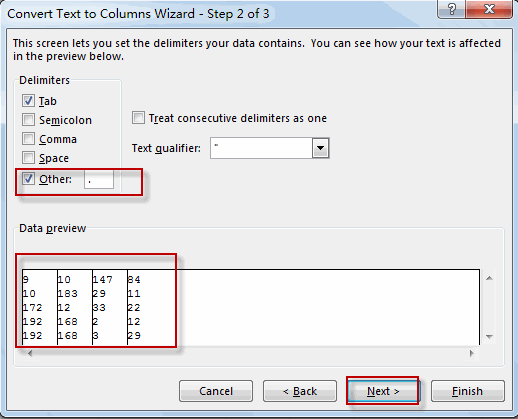#5 select one destination cell to paste the result ( C1). Then click Finsh button.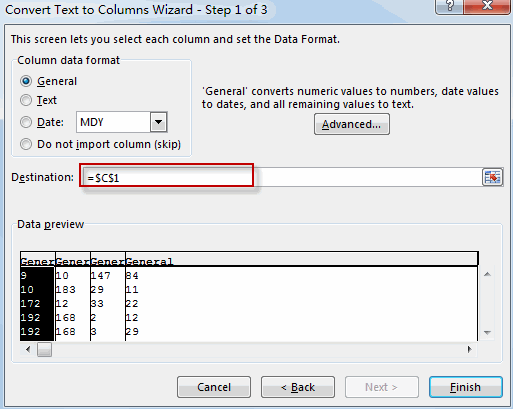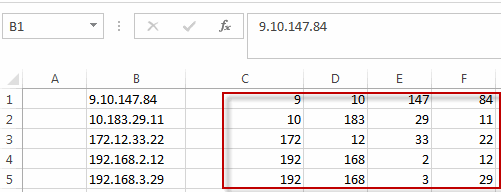#6 select all cells that contain IP addresses.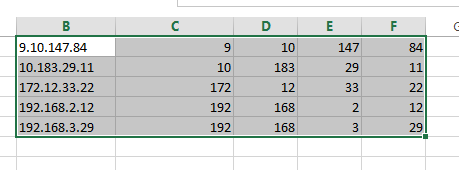#7 click Sort command under Sort&Filter group. The Sort dialog will appear.

#8 clicking Add Level to sort data from column C to F.

#9 click Ok button. You will see that the IP addresses have been sorted.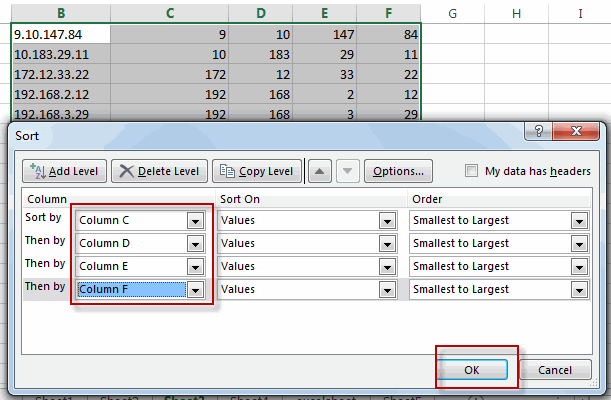### Related Functions

• Excel Text function
The Excel TEXT function converts a numeric value into text string with a specified format. The TEXT function is a build-in function in Microsoft Excel and it is categorized as a Text Function. The syntax of the TEXT function is as below: = TEXT (value, Format code)…
• Excel LEFT function
The Excel LEFT function returns a substring (a specified number of the characters) from a text string, starting from the leftmost character.The LEFT function is a build-in function in Microsoft Excel and it is categorized as a Text Function.The syntax of the LEFT function is as below:= LEFT(text,[num_chars])…t)…
• Excel FIND function
The Excel FIND function returns the position of the first text string (sub string) within another text string.The syntax of the FIND function is as below:= FIND(find_text, within_text,[start_num])…
• Excel RIGHT function
The Excel RIGHT function returns a substring (a specified number of the characters) from a text string, starting from the rightmost character.The syntax of the RIGHT function is as below:= RIGHT (text,[num_chars])…
• Excel MID function
The Excel MID function returns a substring from a text string at the position that you specify.The syntax of the MID function is as below:= MID (text, start_num, num_chars)….